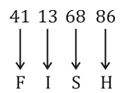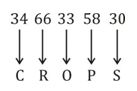# Reasoning Questions Daily Quiz Day – 53

0
117Dear Aspirants, Our SSC Crackers team is providing a new series of Reasoning  Questions for Upcoming Exam so the aspirants can practice it on a daily basis. These questions are framed by our skilled experts after understanding your needs thoroughly. Aspirants can practice these new series questions daily to familiarize with the exact exam pattern and make your preparation effective.

Start Quiz

1) Which answer figure will complete the pattern in the question figure?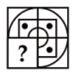a)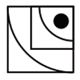b)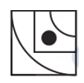c)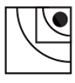d)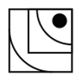2) Which answer figure will complete the pattern in the question figure?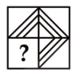a)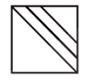b)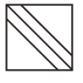c)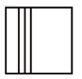d)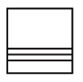3) From the given answer figures, select the one in which the question figure is hidden/embedded.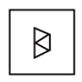a)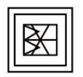b)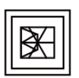c)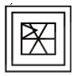d)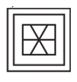4)

From the given answer figures, select the one in which the question figure is hidden/embedded.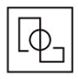a)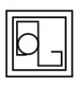b)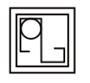c)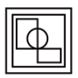d)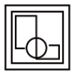5) A piece of paper is folded and punched as shown below in the question figures. From the given answer figures, indicate how it will appear when opened?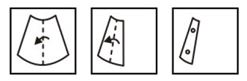a)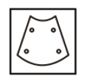b)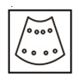c)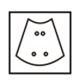d)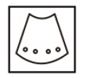6) A piece of paper is folded and punched as shown below in the question figures. From the given answer figures, indicate how it will appear when opened?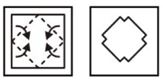a)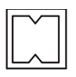b)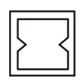c)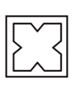d)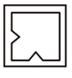7) If a mirror is placed on the line AB, then which of the answer figures is the right image of the given figure?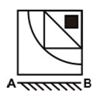a)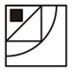b)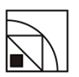c)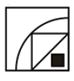d)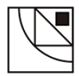8) If a mirror is placed on the line AB, then which of the answer figures is the right image of the given figure?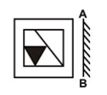a)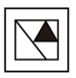b)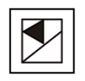c)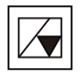d)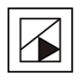9) A word is represented by only one set of numbers as given in any one of the alternatives. The sets of numbers given in the alternatives are represented by two classes of alphabets as shown in the given two matrices. The columns and rows of Matrix-I are numbered from 0 to 4 and that of Matrix-II are numbered from 5 to 9. A letter from these matrices can be represented first by its row and next by its column, for example, ‘L’ can be represented by 11, 42, etc., and ‘A’ can be represented by 65, 96, etc. Similarly, you have to identify the set for the word “FISH”.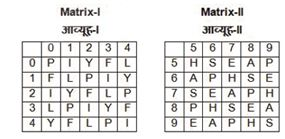a) 66, 00, 68, 14

b) 95, 44, 87, 33

c) 89, 32, 75, 42

d) 41, 13, 68, 86

10) A word is represented by only one set of numbers as given in any one of the alternatives. The sets of numbers given in the alternatives are represented by two classes of alphabets as shown in the given two matrices. The columns and rows of Matrix-I are numbered from 0 to 4 and that of Matrix-II are numbered from 5 to 9. A letter from these matrices can be represented first by its row and next by its column, for example, ‘M’ can be represented by 22, 44, etc., and ‘T’ can be represented by 67, 96, etc. Similarly, you have to identify the set for the word “CROPS”.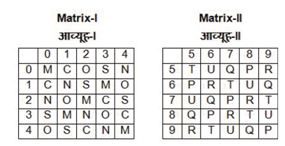a) 13, 68, 10, 67, 40

b) 42, 89, 41, 85, 31

c) 34, 66, 33, 58, 30

d) 13, 56, 24, 95, 14